# Easy, Hands-On Decimal Activities (So Decimals Actually Make Sense!)

If you’re preparing to teach decimals, you may be wondering where even to begin. Decimals can be an abstract, difficult-to-understand concept for some students, and it’s essential to teach them in a way that helps students build their understanding and make sense of what they really mean. In this post, we’ll talk about what decimals are and why we use them (explained in a kid-friendly way that you can use with your students), as well as some effective, hands-on ways to make decimals visual and connect them to fractions. Let’s get started!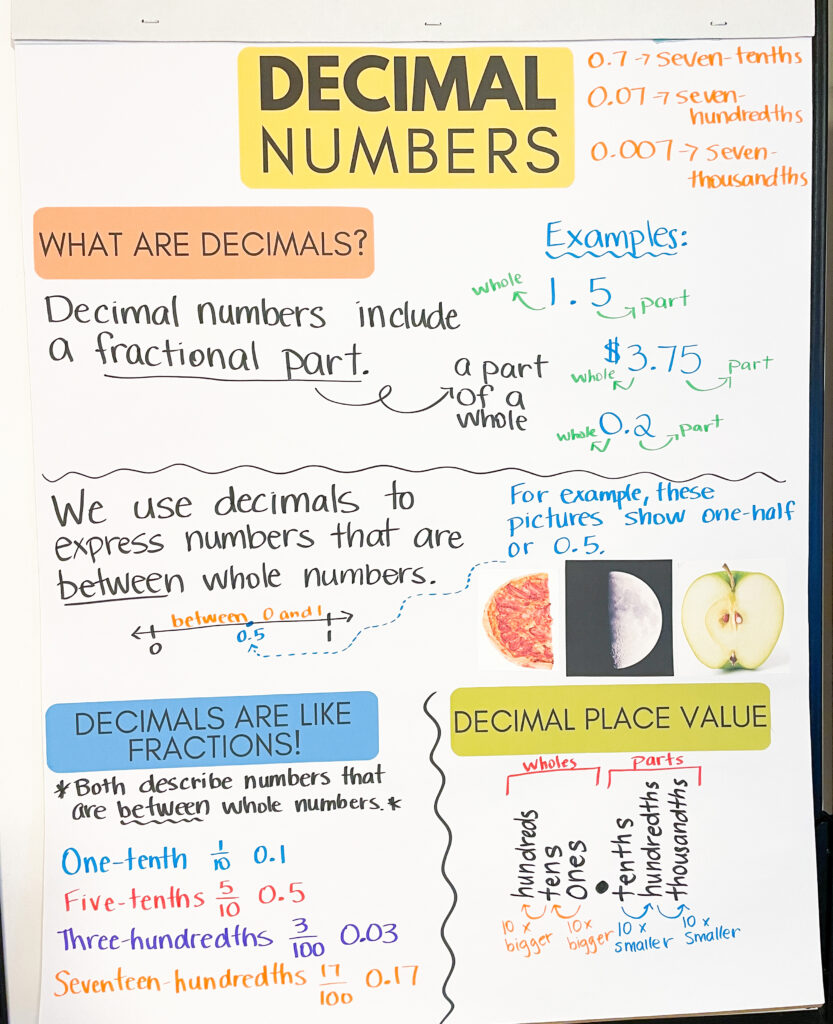## What are Decimals?

A decimal number (sometimes referred to as a ‘decimal’) is a number that includes fractional parts and uses a decimal as a separator between the whole numbers and parts. For example, 1.5 is a decimal number because it refers to one whole and a fractional part (0.5 or one-half), and includes a decimal separator. Decimal numbers are useful because they allow us to describe quantities that are between whole numbers….and this is essential! It is not very often that quantities occur in exact whole numbers. For example…

We probably do not grow EXACTLY 2 inches per year.

The cost of a bag of candy is probably not EXACTLY \$2.00.

Our time on a 200 meter sprint is probably not EXACTLY 1 minute.

We need to have a way to express these “in between” numbers…and decimals are a great solution!

## Decimals as Parts of a Whole

The fractional part (the part that comes after the decimal in a decimal number) is a part of a whole. For example, 0.5 of an apple refers to half of the whole apple. 0.5 of a minute refers to half of a whole minute.

It is important to remember that the amount that the decimal number represents depends on the whole. For example, 0.5 of an apple will look very different than 0.5 of the moon, or 0.5 of a pizza. Or 0.5 of an inch will look very different than 0.5 of a meter. When we are talking about decimals as a quantity, we must understand what our whole is first.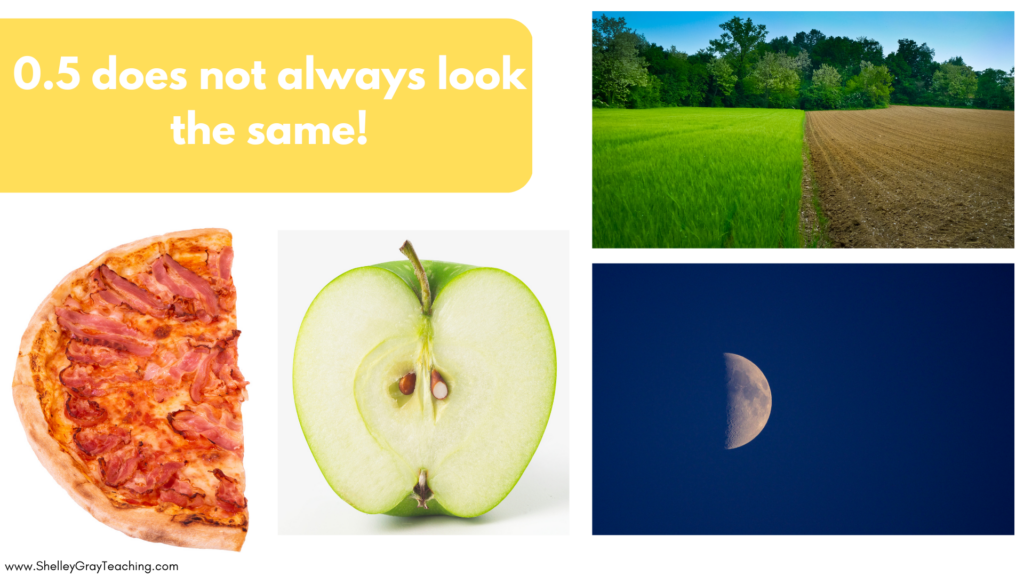## Decimal Place Value: One-Tenth Smaller

It’s important to understand how decimal place value works. As we move farther to the right, we get ten times smaller each time we add a new place. For example, in a number with tenths, hundredths and thousandths, the hundredths are ten times smaller than the tenths. The thousandths are ten times smaller than the hundredths.Confused?

Let’s see this in action.

Here is a whole piece of paper. Let’s cut this paper into tenths. As we can see, each one-tenth piece of paper is ten times smaller than the whole paper.What if we cut these one-tenth pieces into ten pieces? Now each of our new pieces (let’s call them one-hundredths since they are one-hundredth of the whole paper) are ten times smaller than the one-tenth pieces!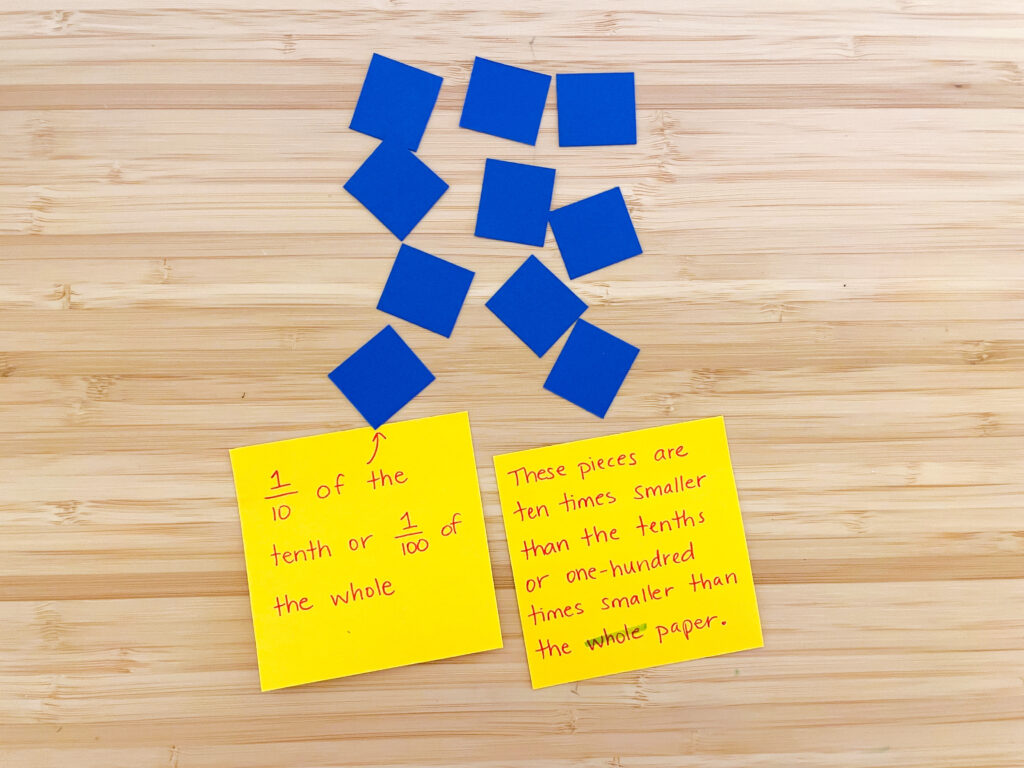If we wanted to show thousandths, we could cut each of our hundredths into ten pieces, since they would be ten times smaller than the hundredths!

## Connecting Decimals To Fractions

If your students have already learned about fractions, fractional parts will be easier to understand. Decimal numbers are like fractions in that they both describe quantities that are in between whole numbers. For example, 1/2 or 0.5 is between 0 and 1.

It’s important to help your students make connections between fractions and decimals. Here are some ideas to do that.

• Teach students about decimal place value. Which place is the tenths place? Which place is the hundredths?
• When you read a decimal number like 0.5, don’t say “zero point five.” Rather, read it as “five-tenths.” This will help students make an automatic connection to the fraction form of 5/10.
• Present numbers in both a fraction and a decimal form when possible. For example, if you are teaching students how to add 0.2 and 0.4, write it as 2/10 + 4/10 as well to help them connect the two.

Another way to help students form connections between fractions and decimals is with hands-on visuals.

## Using Base Ten Blocks to Represent Decimals

Base ten blocks are an amazing (and underused) tool to help students understand decimals. To get started, your students will have to develop some new understanding. Until now, they have probably understood base tens as ones, tens, and hundreds.

But now, since they are older and learning more complex skills, we will use them differently. For the purpose of decimals, we will see these blocks as wholes, tenths, and hundredths. As shown by the picture below, the hundreds block will now represent one whole. The tens block represents one-tenth (because it is one-tenth of the whole or ten times smaller than the whole). The ones block represents one-hundredth because it is one-hundredth of the whole or one-hundred times smaller than the whole.

Your students will need time to develop this new understanding, so be sure to give them lots of time to work with base tens in this new way. Lots of activities are included below!Depending on your curriculum expectations, you could also use the thousands block as the whole, and have the other blocks represent one-tenth (or one-hundred thousandths), one-hundredth (or ten-thousandths), and one-thousandth.

## Base Ten Activities to Reinforce Decimal Number Undertanding

Now that you have helped your students understand base ten blocks as decimal numbers, how can you practice? Here are some activity ideas you can use with the whole class or with small groups.

1. Simply give students a handful of base ten blocks and have them build decimal numbers, draw a representation, and then write them in decimal and fraction form. This is a great introductory activity.
2. Given a decimal number, build it with base ten blocks. Then write it on a mini whiteboard in both decimal and fraction form.
3.  Connect tenths to hundredths. Ask students to prove that, for example, three-tenths is the same as thirty-hundredths.
4. True or False – Present a true or false situation, and have students prove their answer using their blocks. For example, “True or False – 25 hundredths is the same as 2 tenths and 5 hundredths?”
5. Make addition and subtraction with decimal numbers visual. For example, add 0.3 and 0.9. Students will end up with 12 tenths, and then can show the regrouping by trading  ten-tenths for a whole.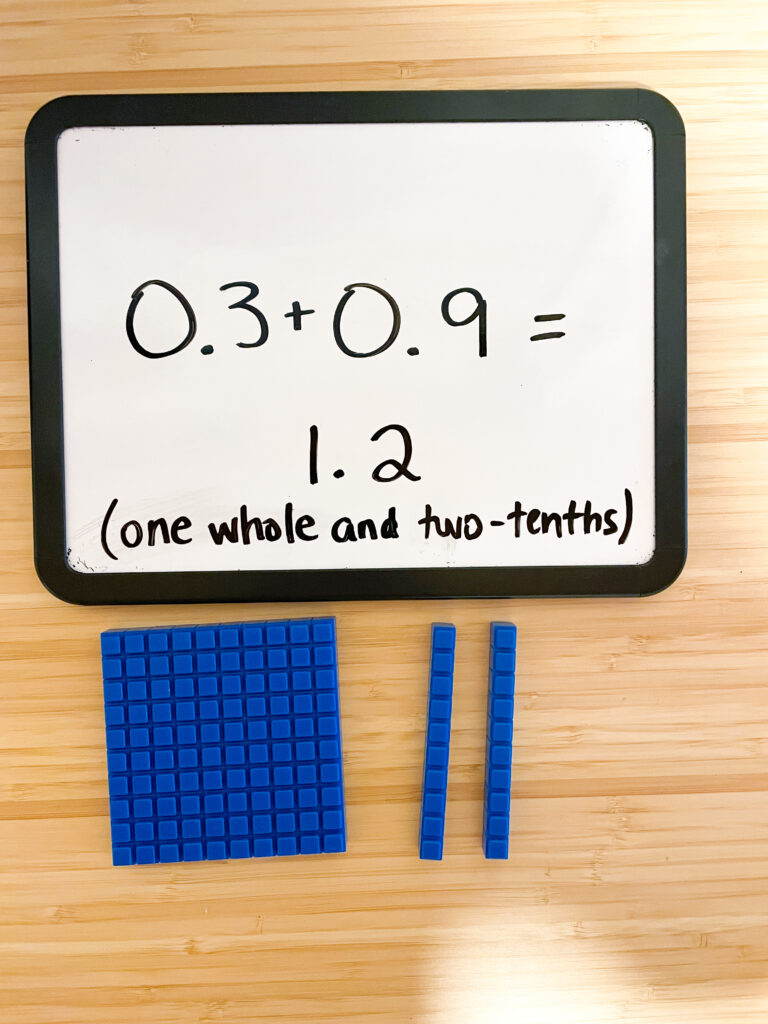Here are some pictures that may spark other ideas for you!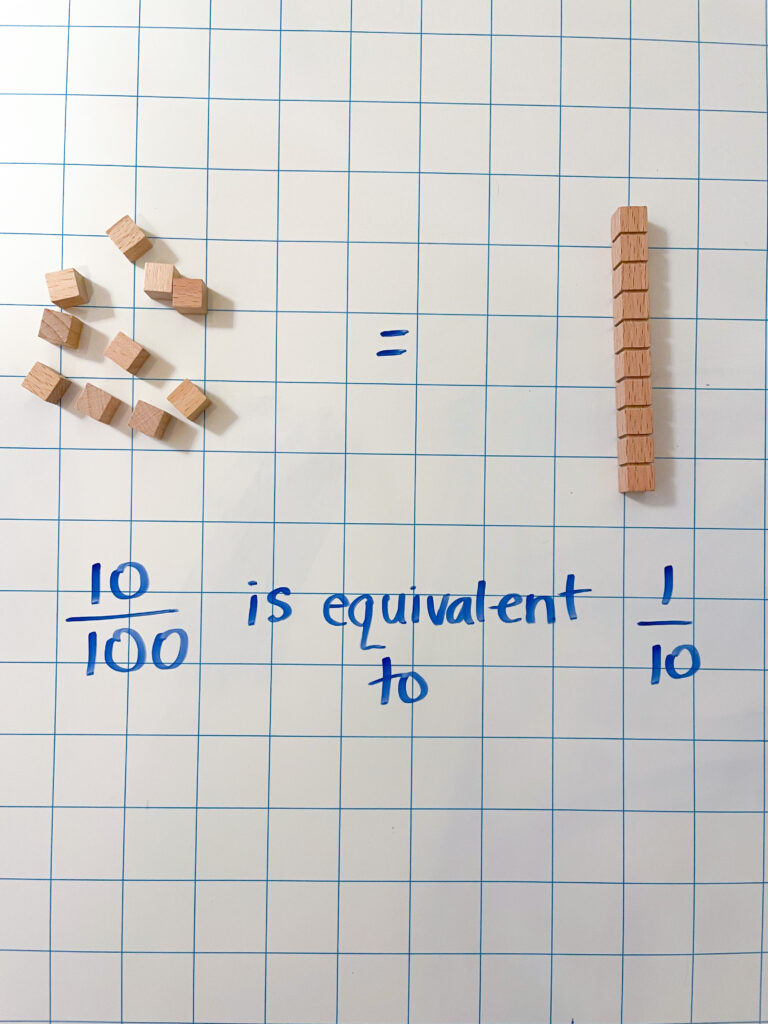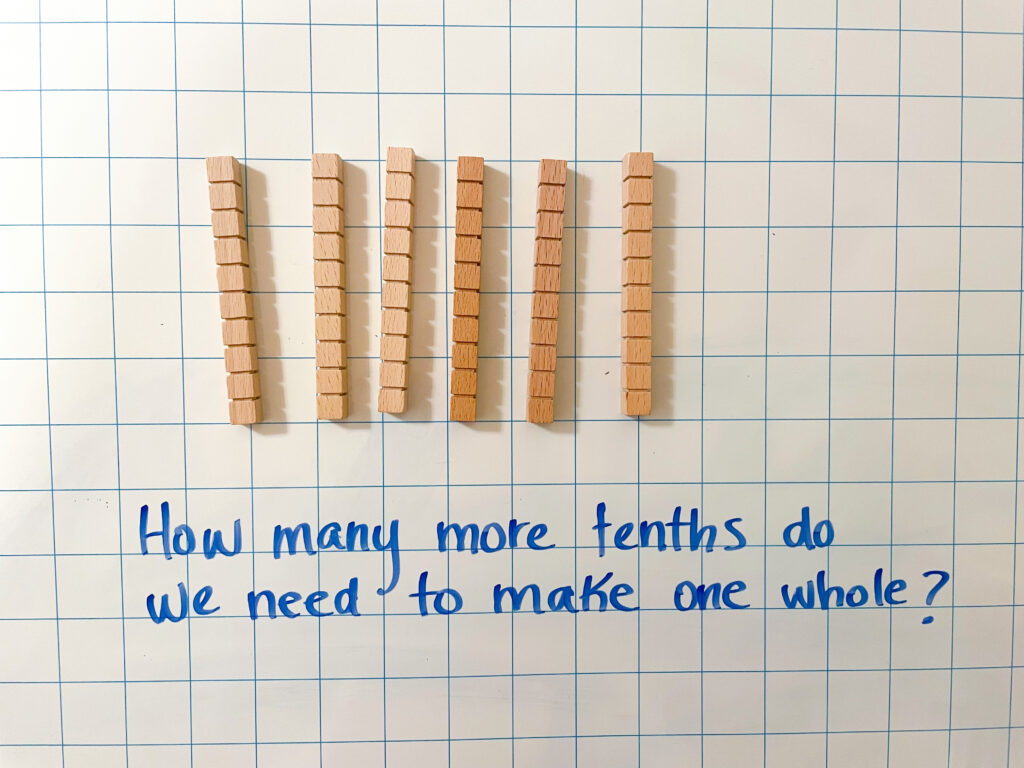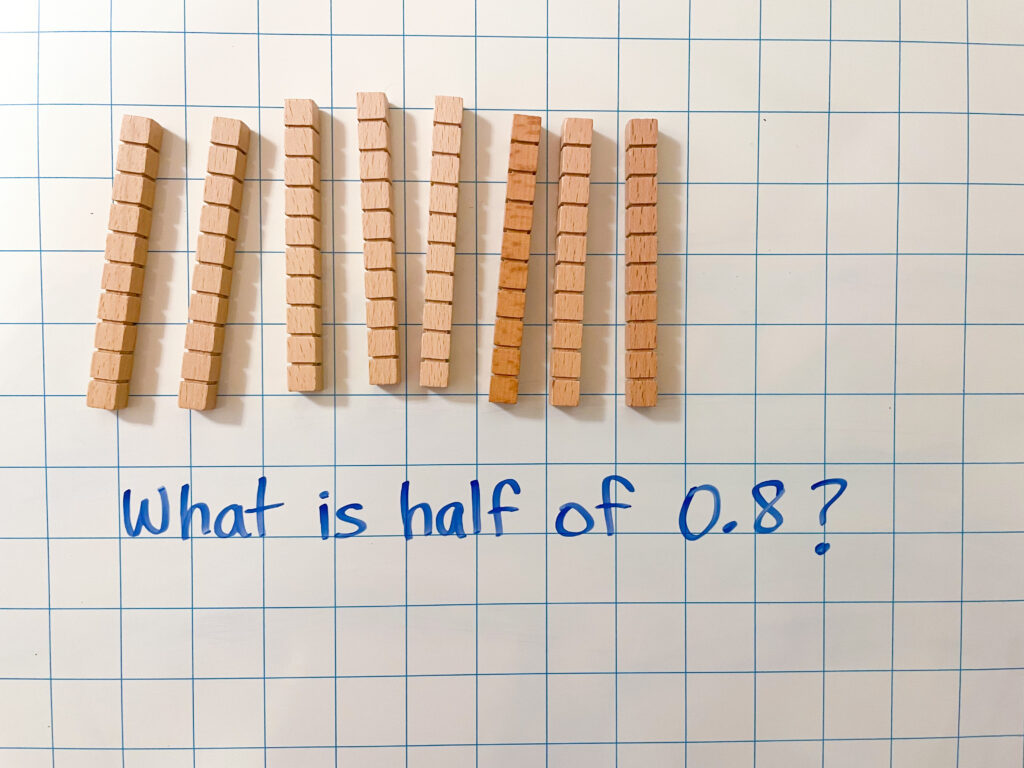## Big Ideas

The big idea here is that we have to explicitly teach decimals (and fractions for that matter) in a hands-on, relevant way that students can really understand. To do this, we need to give opportunities to practice with concrete materials before moving on to abstract representations of decimals. All too often, students begin their decimal experience by simply shading in pictures on a worksheet, and get no concrete or real-life, relevant opportunities. If we want to prevent gaps in their understanding then we must give them these opportunities. Once they have had concrete experiences, and you are confident that they understand what decimals mean, here are some activities you may want to try.

## Resources for Teaching Decimals

### One Comment

•I can’t wait to use this!